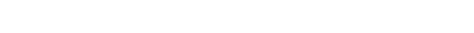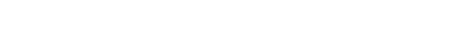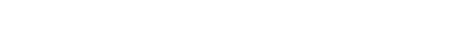# GMAT Quant: Arithmetic with Inequalities

Here are four GMAT Data Sufficiency questions involving inequalities.## The arithmetic of equations: a review

First of all, let’s review what should be more familiar — the arithmetic of equations.   Suppose A = B and P = Q.  The soundbyte is: you can combine them in almost any way imaginable to get a new valid equation.  You can add them, in either order (A + P = B + Q) or (A + Q = B + P).   You can subtract then, either one from the other, in either order (four subtraction equations: e.g. A – P = B – Q).  You can multiply them in either order (A* P = B * Q) or (A * Q = B * P).  You can divide either one by either other (assuming you are not dividing by zero), in either order (four division equations: e.g. A/P = B/Q).  You can raise either one to the power of the other, in either order (four exponentiation equation: e.g. A^P = B^Q).

(Note: for the GMAT, you are responsible for know what happens when you raise a base, say 3, to the power of integers or to the 1/2.  Technically, you can raise any positive base to any power — say, 3 to the pi — but that’s more complicated than the GMAT expects you to know.  For negative bases, things get dicier.  For the GMAT, just worry about negative bases to integer powers, (–2)^3 or (–2)^(–1).   Non-integral powers of negatives leads into complex numbers — again, beyond what the GMAT expects you to know).

Finally, the above may seem simple, and if A & B & P & Q are just individual numbers, then those equations are pretty much “duh”-simple.  Things get considerably more interesting if some or all of those letters are not individual numbers but algebraic expressions.  Even with four different algebra expressions, all this still holds for equations.

Everything gets trickier with inequalities.  First of all, an equation, such as A = B, is inherently symmetrical and “two-sided”, but an inequality is more a one-sided, unidirectional thing.   With any arithmetic of inequalities, we must consider the direction of the inequality.   Furthermore, equations are very intuitive, but some of the arithmetic of inequalities is quite unintuitive.

Adding inequalities is not so bad: you can add inequalities with the same direction of inequality.  Thus, if A > B, and P > Q, then it must be true that A + P > B + Q.  That always works, and it is in many ways what you’d expect.

## Subtracting inequalities

This is the one that’s much trickier.   If A > B, and P > Q, then naïvely one might expect that (A – P) would be greater than (B – Q), but that’s not necessarily the case.  For example, suppose we have 10 > 5 and 2 > 1 — then we could subtract them in the same direction of inequality, and we’d get 8 > 4, which still works.  BUT, suppose 10 > 5, and 100 > 1, both true — now, if we subtract in the same direction of inequality, we find that (–90) is not greater than 4.   If we subtract two inequalities in the same direction of inequality, we may get another true statement, but we are not guaranteed that this will lead to a true statements, so it’s a very unwise move in problem solving.

Here’s the real head-scratcher: we can’t subtract inequalities with the same direction of inequality, but we CAN subtract inequalities with the opposite direction of inequality — in other words, if A > B, and P > Q, then it must be true that (A – Q) > (B – P).  That may be perplexing symbolically, so think about it in real world terms.  Suppose Ann has more money than Bob.  Suppose I take less from the richer person, Ann, and more from the poorer person, Bob.  When I am done, Ann still will have more than Bob — in fact, a greater difference than existed between them before!

## Multiplication and division

Everything gets much hairier with multiplying or dividing inequalities when you consider — one or both sides could be negative.  Hmmm.  If we multiply or divide both sides of inequality by a single negative number, that’s perfectly legal, but we must remember to reverse the direction of inequality.   What happens if we were to multiply or divide inequalities and negatives were involved?  For example, if we know that x > –6 and y > –7, then what can we say about the product xy?  As it turns out, we could pick an x and a y that would satisfy the original inequalities and make the product xy equal absolutely any number on the number line.  This mindboggling bit of math is just to demonstrate why the GMAT is not going to touch multiplying or dividing general inequalities with a ten-foot pole!

Let focus, though, on a special case that could, in very advance problems, appear on the GMAT.  Suppose we know that all four numbers or expressions are positive: A > B > 0 and P > Q > 0.  Then, as with addition, we can multiply inequalities with the same direction: A*P > B*Q must be true.   And, as with subtraction, we can divide inequalities with the opposite direction: A/Q > B/P.  Again, remember the caveat: everything must be positive for these patterns to work.  If anything can be negative, things get much more complicated, so complicated that the GMAT won’t ask about them.

## Absolute value inequalities

Absolute value inequality is a sizeable topic, with some mind-bending twists and turns, but chances are very good the GMAT is not going to probe this topic all that deeply.   In fact, probably most of the absolute value inequalities on the GMAT will be of the form: |x – p|, where p is some given fixed number.

Here, we must remember a few key mathematical facts.   First of all, subtraction gives us distance on the number line.   Technically, subtraction gives signed distance.  What do I mean by that?  Well, 5 – 2 = +3, indicating that it’s a signed distance of positive 3, i.e. three units to the right, from 2 to 5; by contrast, 2 – 5 = –3 indicating that it’s a signed distance of negative 3, i.e. three units to the left, from 5 to 2.

For ordinary distance, distance in the geometric sense of the word, we don’t care about sign or direction — the distance between two points is just a positive number and is the same, whether from A to B or from B to A.   That’s where absolute value comes in.  The expression |p – q| is the distance between numbers p & q on the number line.  That is a HUGE idea.

Thus, |x – 5| is the distance between variable point x and fixed point 5.  The expression |x – 5| < 2 indicates the set of all points x that have a distance to the point 5 of less than two.  Immediately, just thinking about this logic, and without any further calculations, we can see that |x – 5| < 2 is entirely equivalent to 3 < x < 7.   Recognize that this is exactly the kind of math the GMAT adores: with simple logic, you can jump to conclusions without having to do any calculations.  The GMAT goes crazy for math of this sort.   I can’t emphasize enough how important this particular set of logically interconnected ideas is.   If the GMAT asks you anything at all about absolute value inequalities, it is highly likely it would be something of this genre, and unlikely that it would be anything else in this extensive topic.

## Summary

If you had some insights reading this article, I encourage you to take another look at the practice questions at the top before reading the solutions below.   Here’s a similar question from inside Magoosh.

If you have anything to add, or any questions, please let us know in the comment section below.## Solutions to practice problems

1) In this problem, the tempting incorrect answer would be (A), or somehow would involve statement #1 as sufficient, but it’s not.  This gets into an idea discussed in this blog —- we can’t subtract inequalities with the same direction of inequality, but we can subtract inequalities with the opposite direction of inequality.

Statement #1: So the prompt inequality and this statement’s inequality would be true for a + b = 15, c + d = 7, b = 6, d = 2

prompt inequality: 15 > 7  — true

statement #1 inequality: 6 > 2 — true

prompt question: 9 > 5 — a > c, an answer of “yes”

But, both inequalities would still be true for a + b = 20, c + d = 18, b = 15, d = 3

prompt inequality: 20 > 18  — true

statement #1 inequality: 15 > 3 — true

prompt question: 5 < 15 — a < c, an answer of “no”

We can make different choices consistent with all the given statements that would produce either a “yes” or  “no” answer to the prompt question.  This means that, given this statement, we are unable to determine a unique definitive answer to the prompt question.  This statement, alone and by itself, is insufficient.

Statement #2: Here, we are allowed legitimately to subtract the inequalities, because the directions of inequality are in opposite order.  When we subtract (b < d) from (a + b > c + d), we get a > c, a definitive “yes” answer.

Another way to think about it: (a + b) = LARGER, and (c + d) = SMALLER, so of course, the former is greater than the latter.  Now, say that b = tiny, and d = bigger, so of course, b < d.   Now, think about the two differences we will compare:

(i) a = (a + b) – b = LARGER – tiny

(ii) c = (c + d) – d = SMALLER – bigger

Obviously, it start with something LARGER, and subtract something tiny, the result will be greater than starting with something SMALLER and subtracting something bigger.  Therefore, a > c, a definitive answer to the prompt question.

This statement allows us to give a definitive “yes” answer to the prompt, so this statement, alone and by itself, is sufficient.

2) Statement #1: if p = 2 and q = 1, then this statement’s equation is true, 4 > 1, and p > q, so the answer to the prompt is “yes”.

But, if p = –2 and q = –1, then it’s still true that the square of p is larger than the square of q, 4 > 1, but now it’s true that p < q, so the answer to the prompt question is “no.”

We can make different choices consistent with all the given statements that would produce either a “yes” or  “no” answer to the prompt question.  This means that, given this statement, we are unable to determine a unique definitive answer to the prompt question.  This statement, alone and by itself, is insufficient.

Statement #2: if p = 2 and q = 1, then this statement’s equation is true, 8 > 1, and p > q, so the answer to the prompt is “yes”.

Switching to negatives won’t make a difference, because the cube of a negative is still negative.  The values p = –2 and q = –3 satisfy the statement inequality, and it’s still true that p > q

What about fractions?  If p = 1/2 and q = 1/3, then p cubed is still larger than q cubed, (1/8) > (1/27), and p is still greater than q.  No matter what numbers we pick, the inequality in statement #2 directly implies the prompt inequality.  The mathematical way to say this is: cubing, or taking a cube-root, preserves the order of any inequality.

This statement gives us a definitive answer of “yes” to the prompt question, so this statement, alone and by itself, is sufficient.

3)  Think about the distance interpretation of absolute value inequalities.   We want to know: is x further than two units away from 6 on the number line?

Statement #1:  the x-values allowed by this statement are x’s that are more than three units from 4.  Here’s a picture of these values, in green:Notice, the endpoints, 1 & 7, are not allowed, because they are exactly three units from 4, and exactly 3 is not greater than 3.  Most of these points are further than two from the point 6, but some, such as the point 7, is closer than two units to six.  Thus, given this constraint, we could find many points that produce a “yes” answer to the prompt, but some that produce a “no” answer.  Different choices give different answers.   This means that, given this statement, we are unable to determine a unique definitive answer to the prompt question.  This statement, alone and by itself, is insufficient.

Statement #2: the x-values allowed by this statement are x’s that are more than one unit from 8.  Here’s a picture of these values, in green:Again, notice the endpoints are not included.  Many of these points are further than two from the point 6, but some, such as the point 7, is closer than two units to six.  In fact, the point 6 itself — which is a distance of zero units from 6 — is allowed by this statement!  Thus, given this constraint, we could find many points that produce a “yes” answer to the prompt, but some that produce a “no” answer.  Different choices give different answers.   This means that, given this statement, we are unable to determine a unique definitive answer to the prompt question.  This statement, alone and by itself, is insufficient.

Combined statements: Now, we combine the constraints of the individual statements.  Now, the allowed points must be both more than three units from 4 and more than one unit from 8.  Shown in green are the points that simultaneously satisfy both constraints:Now, all the green points are more than two units away from 6, and it absolutely impossible to pick a value of x that simultaneously satisfies the constraints of both statements and is closer than two units to 6.   The combined statements allow for a definitive “yes” answer to the prompt.  Together, the statements are sufficient.

4) Statement #1: this gets at the fundamental meaning of the inequality.   If y is one more than x, it must be greater than x.  To add one to a number means to move it one unit to the right on the number line, so y must be one unit right of x, which means it is greater than x.  This allows us to determine a definite “yes” to the prompt question.   This statement, alone and by itself, is sufficient.

Statement #2: There are a couple ways to think about this one.  One is to treat different categories of numbers.

(i) if x is negative, then by squaring, y will be positive, and y > x.

(ii) if x = 0, y = 1, and y > x

(iii) if x is a fraction between 0 and 1, then its square will also be between 0 and 1, and adding one to this will produce a number greater than 1, between 1 and 2.  Therefore, y > x.

(iv) if x = 1, y = 2, and y > x

(v) if x > 1, then squaring x makes it bigger, and adding one makes that even bigger, so y > x

Thus, for all possible values of x, y > x.  Thus, the prompt gives a definitive “yes” answer.

A totally different way to think about it: using coordinate geometry.  The graph of y = (x^2) is a parabola that passes through the origin.  The graph of y = (x^2) + 1 is this same parabola shift up one in the y-direction, passing through (0, 1) instead of the origin.  Now, compare this shifted parabola to the line y = x.  One of the special properties of the line y = x is that all points above this line, regardless of quadrant, have the property y > x.   Think about the graph:The parabola is always above the line y = x, so every point on the parabola must satisfy y > x.

Either way, this allows us to determine a definite “yes” to the prompt question.   This statement, alone and by itself, is sufficient.

Both statements are individually sufficient.

#### Special Note:

To find out where inequalities sit in the “big picture” of GMAT Quant, and what other Quant concepts you should study, check out our post entitled:

What Kind of Math is on the GMAT? Breakdown of Quant Concepts by Frequency

## Author

•Mike served as a GMAT Expert at Magoosh, helping create hundreds of lesson videos and practice questions to help guide GMAT students to success. He was also featured as "member of the month" for over two years at GMAT Club. Mike holds an A.B. in Physics (graduating magna cum laude) and an M.T.S. in Religions of the World, both from Harvard. Beyond standardized testing, Mike has over 20 years of both private and public high school teaching experience specializing in math and physics. In his free time, Mike likes smashing foosballs into orbit, and despite having no obvious cranial deficiency, he insists on rooting for the NY Mets. Learn more about the GMAT through Mike's Youtube video explanations and resources like What is a Good GMAT Score? and the GMAT Diagnostic Test.